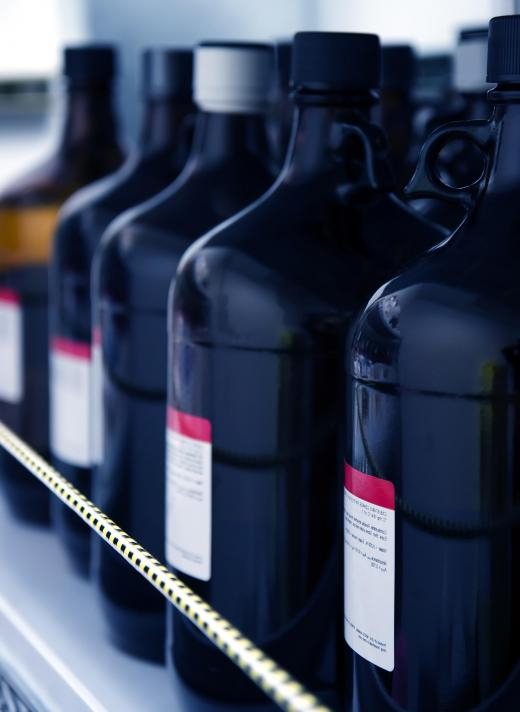Science
Fact Checked

# What is Molar Solubility?

Victoria Blackburn
Victoria Blackburn

Molar solubility (M) is a measure of the ability of a compound, called a solute, to dissolve in a specific substance, called a solvent. Specifically, it is the maximum number of moles of a solute that are able to dissolve in one liter of solvent, so molar solubility is measures as moles/L. When the number of moles dissolved in a liter of solvent is equal to the molar solubility, the solution is said to be saturated, meaning it cannot dissolve more solute.

The value of M relies on a number of variables. Of importance is the solubility product constant, which is designated Ksp. This value is constant for each compound that describes how easily a substance dissolves. Additionally, the ratio of products and reactants in the chemical reaction must be known to calculate the value of M. This is referred to as the stoichiometry of the reaction.The first step to calculating the molar solubility is to balance the chemical equation for dissociation of the substance.

The first step to calculating the molar solubility is to balance the chemical equation for dissociation of the substance. To do this, you must ensure that there are equal numbers of each atom on both the product and reactant side of the chemical reaction. An example of a balanced chemical equation with equal numbers of each atom on the product and reactant sides is shown by the dissociation of a molecule of sodium chloride (NaCl) into sodium (Na) and chlorine (Cl) atoms: NaCl (s) = Na+ (aq) + Cl- (aq). The physical state of the atoms and molecules is designated using s for solid and aq for aqueous or dissolved in water.Molar solubility (M) is a measure of the ability of a compound, called a solute, to dissolve in a specific substance, called a solvent.

To determine M, you will need to look up the Ksp value for the solute, or the starting compound that is being dissolved. This is a measure of the molar solubility of the substance in standard conditions and can be found online or in chemistry textbooks. The following equation describes the relationship between Ksp and the solubility of NaCl: Ksp = [Na][Cl]

Using this equation, you will be able to find the concentrations of the Na and Cl ions in the saturated solution, by taking the square root of the Ksp value. The value of each ion concentration is equivalent to the amount of product that has been dissolved. Therefore, the ion concentration is equal to M.

The value of M depends on a number of factors. Specifically, when the solvent is heated, more energy is available within the system, which allows for greater dissociation of a compound, so molar solubility increases with temperature. If the temperature of a saturated solution is decreased, the M value decreases, and the solute will start to precipitate out of the solution as the temperature decreases. When temperature is decreasing, the concentration of the solution before it begins to precipitate is larger than M, and such a solution is said to be supersaturated.

## You might also Like